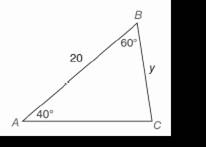Chapter 11.CR, Problem 11CRElementary Geometry For College St...

7th Edition
Alexander + 2 others
ISBN: 9781337614085

Solutions

Chapter
SectionElementary Geometry For College St...

7th Edition
Alexander + 2 others
ISBN: 9781337614085
Textbook Problem

In Review Exercises 9 to 12, use the Law of Sines or the Law of cosines to find the indicated length of side or angle measure. Angle measures should be found to the nearest degree; distances should be found to the nearest tenth of a unit.To determine

To find:

The indicated length of side or angle measure using the law of sines or the Law of cosines.

Explanation

Procedure used:

In any triangle ABC such that

AB=c,

BC=a,

CA=b,

mA=α,

mB=β, and

mC=γ.

The Law of cosines is given by

a2=b2+c2-2bccosα

b2=c2+a2-2cacosβ

c2=a2+b2-2abcosγ

The Law of sines is given by

asinα=bsinβ=csinγ

Calculation:

Given:

From the above figure we have

a=y,

c=20,

α=40°, and

β=60°

By the law of sine we have

asinα=bsinβ=csinγ

Let us find the value of γ by the angle sum property of a triangle. In any triangle, the sum of the all the three angles is 180°

(i.e

Still sussing out bartleby?

Check out a sample textbook solution.

See a sample solution

The Solution to Your Study Problems

Bartleby provides explanations to thousands of textbook problems written by our experts, many with advanced degrees!

Get Started

In Exercises 7-28, perform the indicated operations and simplify each expression. 17. 1+1x11x

Applied Calculus for the Managerial, Life, and Social Sciences: A Brief Approach

Solve the equations in Exercises 126. (x2+1)5(x+3)4+(x2+1)6(x+3)3=0

Finite Mathematics and Applied Calculus (MindTap Course List)

Prove the statement using the , definition of a limit. limx10(345x)=5

Single Variable Calculus: Early Transcendentals, Volume I

Perform the indicated operation for the following. 15,000188

Contemporary Mathematics for Business & Consumers

For f(x) = x2 and g(x) = 2x + 1, (f g)(x), = a) 2x2 + 1 b) (2x)2 + 1 c) (2x + 1)2 d) x2(2x + 1)

Study Guide for Stewart's Single Variable Calculus: Early Transcendentals, 8th

The area of the shaded region is given by:

Study Guide for Stewart's Multivariable Calculus, 8th